### Home > MC2 > Chapter 1 > Lesson 1.2.4 > Problem1-93

1-93.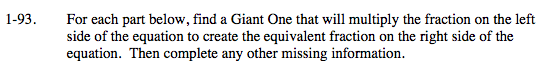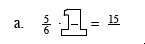If the desired numerator is 15, what must you multiply 5 by to get this number? The number you find is the numerator for the 'one' multiplier.

Now try using the multiplicative identity. Since the number is multiplied by 'one' to create an equivalent fraction, what must the denominator of the 'one' multiplier be?

Multiply denominators to solve for the missing number.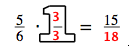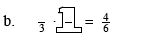Refer to part (a).

Instead of having the entire original fraction, you are given the entire equivalent fraction. Use the same strategy from part (a), but instead work backwards with division after finding the 'one' multiplier.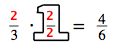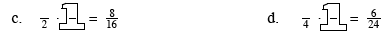Now, try solving for part (c) and (d) using a similar method.